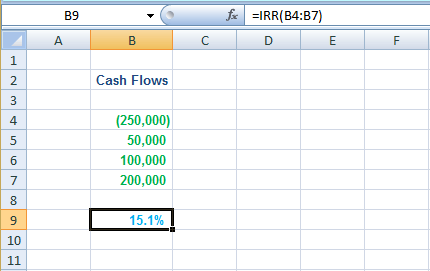# Internal Rate of Return (IRR)

## Definition

Internal Rate of Return, often simply referred to as the IRR, is the discount rate that causes the net present value of future cash flows from an investment to equal zero.

## Formula

Internal Rate of Return:

=  R1  +  [ (NPV1 x (R2 – R1)% ÷ (NPV1 – NPV2) ]

Where:

R1      =   Lower discount rate

R2      =   Higher discount rate

NPV1   =   Higher Net Present Value (derived from R1)

NPV2   =   Lower Net Present Value (derived from R2)

## Explanation

IRR represents the intrinsic rate of return that is expected to be derived from an investment considering the amount and timing of the associated cash flows.

For example, an IRR of 10% suggests that the proposed investment will generate an average annual rate of return equal to 10% over the life of the project taking into consideration the amount and timing of the expected cash inflows and outflows specific to that investment.

IRR is derived by extrapolating 2 net present values that have been calculated using 2 random discount rates.

The calculation and interpretation of IRR can be simplified into the following 4 Steps:

## Calculation

Step 1: Select 2 discount rates for the calculation of NPVs

You can start by selecting any 2 discount rates on a random basis that will be used to calculate the net present values in Step 2.

It is important however not to select discount rates that are ridiculously distant from the IRR (e.g. 10% and 90%) as it could undermine accuracy.

Although guessing the IRR before you have calculated it would be kind of hard, try your best to keep the two discount rates that you select within a reasonable range to improve the accuracy of your calculation.

Step 2: Calculate NPVs of the investment using the 2 discount rates

You shall now calculate the net present values of the investment on the basis of each discount rate selected in Step 1.

Step 3: Calculate the IRR

Using the 2 discount rates from Step 1 and the 2 net present values derived in Step 2, you shall calculate the IRR by applying the IRR Formula stated above.

Step 4: Interpretation

The decision rule for IRR is that an investment should only be selected where the cost of capital (WACC) is lower than the IRR.

The decision rule above will lead to the same conclusion as the NPV analysis where only one investment is being considered.

Where multiple investments are being considered, IRR should not be used as the primary appraisal tool because NPV analysis provides a better measure of the impact of different projects on the shareholder wealth. IRR should still be used, however, as a risk assessment tool to measure the sensitivity of different investment options towards the cost of capital.

## Example

Mr. A is considering investing \$250,000 in a business.

The cost of capital for the investment is 13%.

Following cash flows are expected from the investment:

Year                          \$
0                       (250,000)
1                          50,000
2                        100,000
3                        200,000

## Solution

Step 1: Select 2 discount rates for the calculation of NPVs

We can take 10% (R1) and 20% (R2) as our discount rates.

Step 2: Calculate NPVs of the investment using the 2 discount rates

Net Present Value @ 10%

Cash Flow Discount Factor Present Value

A

B

A x B

(250,000)

1.000

(250,000)

50,000

0.909

45,450

100,000

0.826

82,600

200,000

0.751

150,200

NPV1

28,250

Net Present Value @ 20%

Cash Flow Discount Factor Present Value

A

B

A x B

(250,000)

1.000

(250,000)

50,000

0.833

41,650

100,000

0.694

69,400

200,000

0.579

115,800

NPV2

-23,150

Step 3: Calculate the IRR

Internal Rate of Return:

=  R1%  +   [(NPV1 x (R2 – R1)%) ÷ (NPV1 – NPV2)]

=  10%  +   [(28,250 x (20 – 10)%) ÷ (28,250 – (- 23,150))]

=  10%  +   [(28,250 x 10%) ÷ (28,250 + 23,150)]

=  10%  +   5.5%

=  15.5%

Note:

A more accurate IRR can be calculated using the Microsoft Excel’s IRR function. Simply write the cash flows of the investment in separate cells and define the range of those cells in the IRR function as below:As you can see, the IRR function returns a value of 15.1% which varies slightly from the manual calculation above.

Step 4: Interpretation

The investment should be accepted by Mr. A because the cost of capital (i.e. 13%) is lower than the IRR of 15.5%.

The cost of capital will need to increase by more than 19.2%* for the investment to become financially nonviable.

* (15.5% – 13%) ÷  13% = 19.2%

• IRR is expressed in percentage terms which is often easier to understand for people from non-financial background;
• Helps in the assessment of sensitivity of an investment towards changes in cost of capital.

## Limitations

• May not lead to the optimum decision where multiple investment options are being considered (e.g. investment with the highest IRR is selected instead of investment that will generate the highest net present value). NPV analysis remains the most effective investment appraisal tool in this regard.
• Multiple IRRs can exist for the same investment where the timing of cash outflows is unusual. Interpreting IRR can be tricky in such scenarios.
• The calculation of IRR assumes that the cash inflows earned during the project life are ‘re-invested’ at the rate of the IRR. As the rate of IRR is usually higher than the cost of capital, some financial experts argue that a more prudent assumption would be to re-invest the cash inflows at the rate of the cost of capital. This forms the basis for the development of Modified Internal Rate of Return (MIRR).